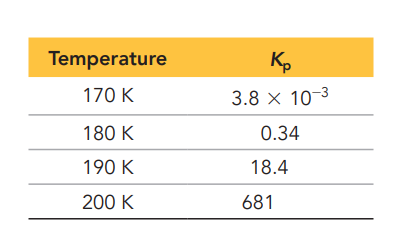×
Get Full Access to Chemistry: A Molecular Approach - 5 Edition - Chapter 19 - Problem 80
Get Full Access to Chemistry: A Molecular Approach - 5 Edition - Chapter 19 - Problem 80

×ISBN: 9780134874371 2047

## Solution for problem 80 Chapter 19

Chemistry: A Molecular Approach | 5th Edition

• Textbook Solutions
• 2901 Step-by-step solutions solved by professors and subject experts
• Get 24/7 help from StudySoup virtual teaching assistantsChemistry: A Molecular Approach | 5th Edition

4 5 1 381 Reviews
11
0
Problem 80

Consider the reaction:

$$2 \mathrm{NO}(g)+\mathrm{O}_{2}(g) \rightleftharpoons 2 \mathrm{NO}_{2}(g)$$

The following data show the equilibrium constant for this reaction measured at several different temperatures. Use the data to find $$\Delta H_{\mathrm{rxn}}^{\circ}$$ and $$\Delta S_{\mathrm{rxn}}^{\circ}$$ for the reaction.Text Transcription:

2 NO( g) + O2( g) rightleftharpoons 2 NO2( g)

Delta H_rxn^circ

Delta S_rxn^circ

Step-by-Step Solution:
Step 1 of 3

ACIDS AND BASES Acids: produces H+ (which is the same as protons and hydronium ion, H3O+) AND are mostly covalent compounds! H+ + OH- à H2O acid base neutralization The “protics” HCl à H+ Monoprotic ** Compounds that are monoprotic and H2SO 4à 2 H+ Diprotic diprotic are strong acids! H3PO 4à 3 H+ triprotic ** Triprotic are weak acids Ex: H2CO3 à Diprotic weak acid CH 3COOH à monoprotic weak acid Characteristics of acids: Sour Turns blue litmus paper into pink Strong Acids v. Weak acids : 2 converting pH concentration 3 Bases: substances producing OH- in an aqueous solutions AND are ALL ionic compounds Characteristics: Taste is unknown (no one has actually tried it since it is dangerous Turns pink litmus paper into blue Indicators: work in different pH ranges 4 Ex: phenolphthalein detects the pH between 6-8 Bromocresolblue deteacts pH between 3-5 Buffers: Maintain small changes of pH Weak acids/ conjugate bases Weak bases / conjugate acids 5

Step 2 of 3

Step 3 of 3

##### ISBN: 9780134874371

The answer to “?Consider the reaction:$$2 \mathrm{NO}(g)+\mathrm{O}_{2}(g) \rightleftharpoons 2 \mathrm{NO}_{2}(g)$$The following data show the equilibrium constant for this reaction measured at several different temperatures. Use the data to find $$\Delta H_{\mathrm{rxn}}^{\circ}$$ and $$\Delta S_{\mathrm{rxn}}^{\circ}$$ for the reaction. Text Transcription:2 NO( g) + O2( g) rightleftharpoons 2 NO2( g)Delta H_rxn^circDelta S_rxn^circ” is broken down into a number of easy to follow steps, and 47 words. The full step-by-step solution to problem: 80 from chapter: 19 was answered by Aimee Notetaker, our top Chemistry solution expert on 06/03/22, 04:20PM. This full solution covers the following key subjects: . This expansive textbook survival guide covers 24 chapters, and 162 solutions. Chemistry: A Molecular Approach was written by Aimee Notetaker and is associated to the ISBN: 9780134874371. This textbook survival guide was created for the textbook: Chemistry: A Molecular Approach, edition: 5. Since the solution to 80 from 19 chapter was answered, more than 201 students have viewed the full step-by-step answer.

## Discover and learn what students are asking

Calculus: Early Transcendental Functions : Introduction to Functions of Several Variables
?In Exercises 7-18, find and simplify the function values. $$g(x, y)=\int_{x}^{y} \frac{1}{t} d t$$ (a) (4,1) (b) (6,3)

Calculus: Early Transcendental Functions : Second-Order Homogeneous Linear Equations
?Finding a General Solution In Exercises 5-30,find the general solution of the linear differential equation. y’’ + 2y' = 0

Statistics: Informed Decisions Using Data : Tests for Independence and the Homogeneity of Proportions
?Credit Risk Traditional underwriting to determine the risks associated with lending include credit scores, income, and employment history. The online

Unlock Textbook Solution